# Scaling and Correspondence Problems

Scaling and Correspondence Problems• There are 3 red marbles in each bag and we need 30 red marbles in total.
• To work out how many bags we need, we divide by the number of red marbles in each bag.
• We divide because we want 30 marbles which are shared into equal groups of three.
• 30 ÷ 3 = 10 and so we need 10 bags.
• If we had ten bags, then we would have ten lots of 3 red marbles.
• 10 × 3 = 30.

We divide the amount we need by the amount in each group to work out how many groups we need.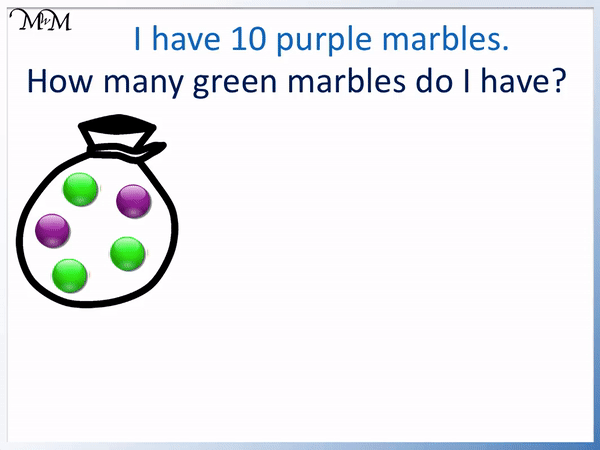• We need to find out how many bags of marbles we need to get 10 purple marbles altogether.
• We divide 10 by the number of purple marbles in each bag to find the number of bags we need.
• 10 ÷ 2 = 5 and so, we must have five bags.
• In each bag there are 3 green marbles.
• Five bags each containing 3 green marbles is 5 lots of 3.
• 5 × 3 = 15 and so there are 15 green marbles in total.# Scaling and Correspondence Problems

## What is a Correspondence Problem?

Correspondence problems are also known as integer scaling problems. In the English national curriculum for Year 4, we are asked to ‘solve harder correspondence problems such as n objects are connected to m objects’.

Correspondence problems involve multiplying or dividing groups of amounts that are in given ratios.

Some examples of correspondence problems would be:

• “I buy pens in packets containing 2 blue and 5 red pens. If I buy 20 blue pens, how many red pens do I have?”
• “For every 3 white chocolates in a box, there are 2 dark. If I have 6 dark chocolates, how many chocolates do I have in total?”

These problems involve working out how many groups, packets or boxes we have in total and then using this to work out the missing number needed.

## How to Solve Correspondence Problems with n Objects Connected to m Objects

To solve correspondence problems, first work out the total number of groups using division and secondly, multiply to find the missing amount.

• Divide the total amount of the first object by the amount of these in each group.
• Multiply the number of the required item in each group by the number of groups.

In the example below we have three bags of marbles each containing 1 blue and 3 red marbles. We want to know how many blue marbles this is in total.

We already know that we have three bags in total, so we do not need to work this out.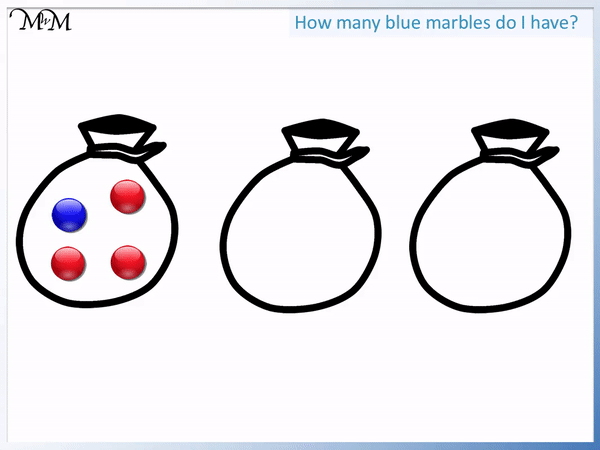We have 3 groups and each group contains 1 blue marble.

We have 3 lots of 1 blue marble. We have 3 × 1 = 3.

We wanted to know how many blue marbles there are and so we multiplied the number of blue marbles in each bag by the total number of bags.

We multiply the number of the required item in each group by the number of groups.

In this next example we still have the same 3 bags of marbles, however this time we need to find the total number of red marbles.

We multiply the number of the required item in each group by the number of groups.

The required item is red marbles and so we multiply the number of red marbles in each bag by the total number of bags.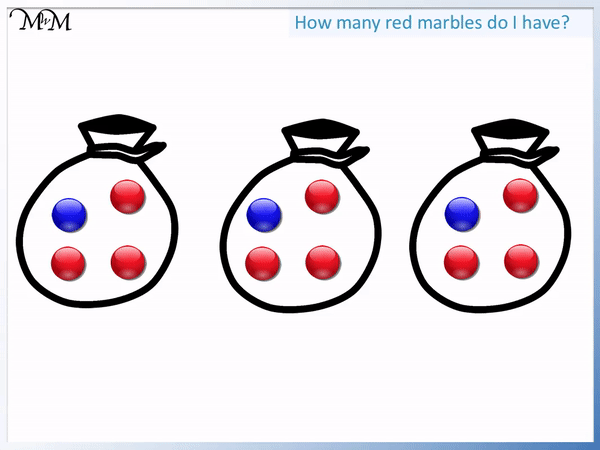In both of these examples, we already knew the total number of groups. We knew that we had 3 bags.

In the next scaling problem we have an example where we first need to calculate the number of groups.

We buy bags of marbles that contain 1 blue and 3 red. However, we need 30 red marbles in total and are asked how many blue marbles we would have if we did so.

The first step is to find the number of bags required to give us 30 red marbles.

We can think of this as 30 red marbles shared equally into groups of 3 because there are 3 red marbles in each group.

In Mathematics, we use the divide operation to share equally.We have 30 red marbles shared equally into groups of 3.

This is 30 ÷ 3 = 10. There are 10 bags of marbles.

We know that if we had 10 bags, each containing 3 red marbles then this would give us 30 red marbles in total.

Now that we know that we have 10 bags, we can find how many blue marbles this would be. There is 1 blue marble in each bag.

10 lots of 1 is 10 and so, there would be 10 blue marbles if we bought 30 reds.

Alternatively, we could see that in the given ratio, we have 3 reds to 1 blue. There are three times more red marbles than blue and so, we can just divide the amount of red marbles by 3 to find the number of blue.

If we had 30 red, then dividing this by 3 gives us 10 blue marbles.

In this next example we have the same bag containing 1 blue and 3 red marbles.

We know we have 12 red marbles and are asked how many blue marbles we must have.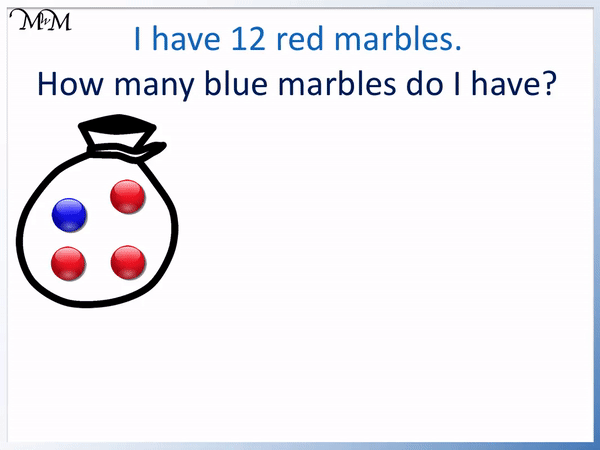Again we can divide 12 red marbles by 3 to find that we have 4 bags in total.

In 4 bags, there are 4 lots of 1 blue marble, which gives 4 blue marbles in total.

Alternatively we know that no matter how many red marbles there are, we can divide this by 3 to find the number of blue marbles.

12 ÷ 3 = 4.

Here is an example of a scaling problem with a different ratio of marbles.

We have 2 purple to 3 green marbles in each bag. We have 6 greens in total and need to find out how many bags there are so that we can find how many purple marbles we have.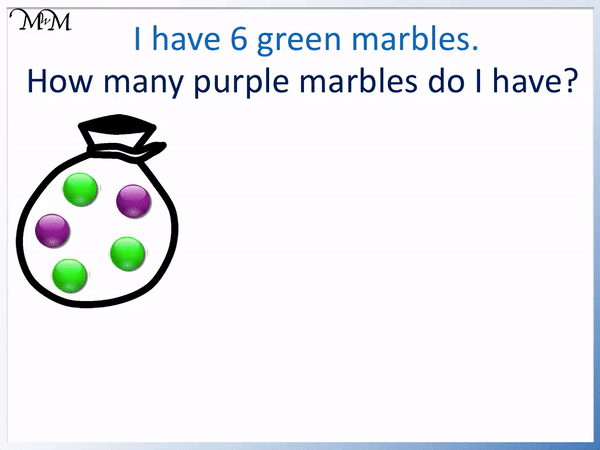To find the number of bags we divide the number of green marbles in total by the number of green marbles in each bag.

6 ÷ 3 = 2 and so, we have 2 bags.

Each bag contains 2 purple marbles and so, we have 2 bags each containing 2 purple marbles. 2 lots of 2.

2 × 2 = 4 and so, we have 4 purple marbles if we have 6 green.

In the next correspondence problem, we have the same bag of marbles but are asked how many green marbles we have if we have 10 purple marbles.

We first find the number of bags by dividing the 10 purple marbles by the number of purple marbles in each bag.There are 2 purple marbles in every bag.

10 ÷ 2 = 5 and so, there are 5 bags in total.

Now that we know that we have 5 bags, we can multiply this by the number of green marbles in each bag, which is 3.

5 × 3 = 15. We have 15 green marbles in total.

In this example we have packets of pencils, each containing 2 blue and 4 green.

We are asked how many green pencils we have if we buy 6 packets.

Since we already know that we have 6 packets, we simply multiply 6 by the number of green pencils in each packet.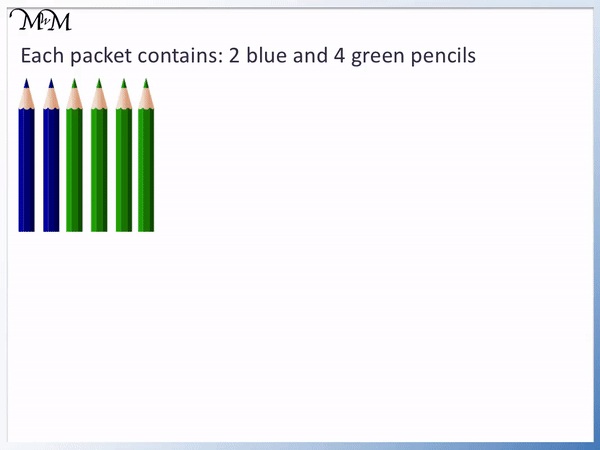There are 4 green pencils in each packet and so, we have 6 lots of 4.

6 × 4 = 24. We have 24 green pencils in 6 packets.

In this next correspondence problem, we have 40 green pencils in total and are asked how many blue pencils we have.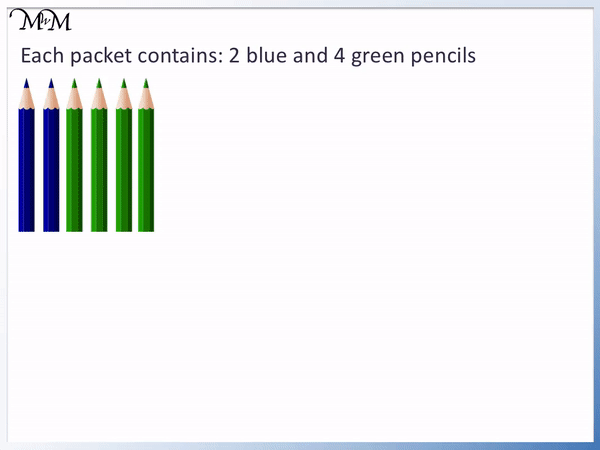40 ÷ 4 = 10 and so there are ten packets in total.

In ten packets, there are 20 green pencils since there are 2 in each packet.

Alternatively we can solve this scaling problem using the ratio of pencils in each packet.

In each packet there are 2 blue and 4 green. 2 is half of 4. There are half as many blue pencils as there are green pencils.

If we have 40 green pencils, then we have half this number of blue pencils.

Half of 40 is 20.

In this next problem we know that we have 8 blue pencils. There are 2 blue pencils in each packet and so we divide 8 by 2 to find the number of packets required.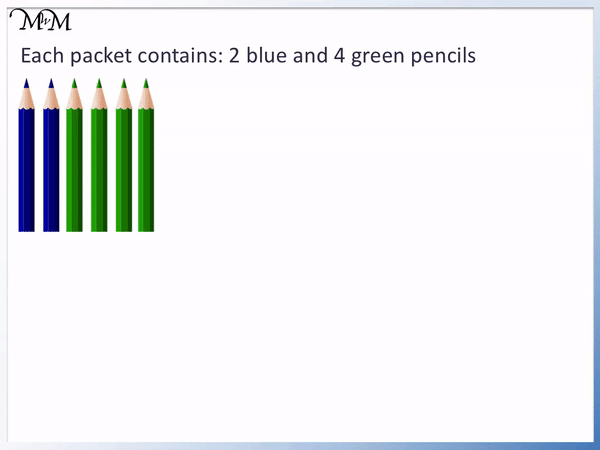8 ÷ 2 = 4 and so there must be 4 packets.

We are asked how many pencils there are in total of both colours.

There are 2 blue and 4 green in each packet. We have 2 + 4 = 6 pencils in each packet.

We have 4 packets, each containing 6 pencils. 4 lots of 6.

4 × 6 = 24 and so, we have 24 pencils in 6 packets.

In this next scaling problem, we have a box of chocolates containing 2 strawberry, 1 dark, 3 mint and 2 caramel.

We buy 6 of these boxes and are asked how many mint chocolates this would be.We have 6 lots of 3 mint chocolates.

6 × 3 = 18 and so, we have 18 mint chocolates in 6 boxes.

In this correspondence problem, we have 14 strawberry chocolates and are asked how many chocolates we have in total of all flavours.

We need to work out the number of boxes and then multiply this amount by the number of chocolates in each box.There are 2 strawberry chocolates in each box, so to work out the total number of boxes, we divide 14 by 2.

14 ÷ 2 = 7 and so we have 7 boxes. 7 boxes, each containing 2 strawberry chocolates must give us the 14 strawberry chocolates required.

We now calculate the number of chocolates in total in 7 boxes.

The number of chocolates in each box is the sum of the strawberry, dark, mint and caramel flavours.

In each box there are 2 + 1 + 3 + 2 chocolates. There are 8 chocolates in each box.

We have 7 boxes, each containing 8 chocolates. 7 lots of 8.

7 × 8 = 56 and so there are 56 chocolates in seven boxes.Now try our lesson on Addition and Subtraction Word Problem Keywords where we learn how to solve word problems.